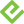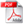# Microsoft Excel Data Analysis and Business Modeling, 5th Edition

### eBook (Watermarked)

• List Price: \$39.99
• Includes EPUB, MOBI, and PDF
• This eBook includes the following formats, accessible from your Account page after purchase:EPUB The open industry format known for its reflowable content and usability on supported mobile devices.MOBI The eBook format compatible with the Amazon Kindle and Amazon Kindle applications.PDF The popular standard, used most often with the free Adobe® Reader® software.

This eBook requires no passwords or activation to read. We customize your eBook by discreetly watermarking it with your name, making it uniquely yours.

Also available in other formats.

## Description

• Dimensions: 7-3/8" x 9"
• Edition: 5th
• eBook (Watermarked)
• ISBN-10: 1-5093-0425-8
• ISBN-13: 978-1-5093-0425-7

Master business modeling and analysis techniques with Microsoft Excel 2016, and transform data into bottom-line results. Written by award-winning educator Wayne Winston, this hands on, scenario-focused guide helps you use Excel’s newest tools to ask the right questions and get accurate, actionable answers. This edition adds 150+ new problems with solutions, plus a chapter of basic spreadsheet models to make sure you’re fully up to speed.

• Quickly transition from Excel basics to sophisticated analytics
• Summarize data by using PivotTables and Descriptive Statistics
• Use Excel trend curves, multiple regression, and exponential smoothing
• Master advanced functions such as OFFSET and INDIRECT
• Delve into key financial, statistical, and time functions
• Leverage the new charts in Excel 2016 (including box and whisker and waterfall charts)
• Make charts more effective by using Power View
• Tame complex optimizations by using Excel Solver
• Run Monte Carlo simulations on stock prices and bidding models
• Work with the AGGREGATE function and table slicers
• Create PivotTables from data in different worksheets or workbooks
• Learn about basic probability and Bayes’ Theorem
• Automate repetitive tasks by using macros

Follow the instructions to download this book's companion content. Part 1 includes files from Chapters 1-45; Part 2 contains files from Chapters 46-89.

2. If prompted, click Save.
3. Locate the .zip file on your computer. Right-click the file, click Extract All, and then follow the instructions.

## Sample Content

Chapter 2 Range names

Chapter 3 Lookup functions

Chapter 4 The INDEX function

Chapter 5 The MATCH function

Chapter 6 Text functions

Chapter 7 Dates and date functions

Chapter 8 Evaluating investment by using net present value criteria

Chapter 9 Internal rate of return

Chapter 10 More Excel financial functions

Chapter 11 Circular references

Chapter 12 IF statements

Chapter 13 Time and time functions

Chapter 14 The Paste Special command

Chapter 15 Three-dimensional formulas and hyperlinks

Chapter 16 The auditing tool

Chapter 17 Sensitivity analysis with data tables

Chapter 18 The Goal Seek command

Chapter 19 Using the Scenario Manager for sensitivity analysis

Chapter20The COUNTIF, COUNTIFS, COUNT, COUNTA, and COUNTBLANK functions

Chapter 21 The SUMIF, AVERAGEIF, SUMIFS, and AVERAGEIFS functions

Chapter 22 The OFFSET function

Chapter 23 The INDIRECT function

Chapter 24 Conditional formatting

Chapter 25 Sorting in Excel

Chapter 26 Tables

Chapter 27 Spin buttons, scroll bars, option buttons, check

boxes, combo boxes, and group list boxes

Chapter 28 The analytics revolution

Chapter 29 An introduction to optimization with Excel Solver

Chapter 30 Using Solver to determine the optimal product mix

Chapter 31 Using Solver to schedule your workforce

Chapter 32 Using Solver to solve transportation or distribution problems

Chapter 33 Using Solver for capital budgeting

Chapter 34 Using Solver for financial planning

Chapter 35 Using Solver to rate sports teams

Chapter 36 Warehouse location and the GRG Multistart and Evolutionary Solver engines

Chapter 37 Penalties and the Evolutionary Solver

Chapter 38 The traveling salesperson problem

Chapter 39 Importing data from a text file or document

Chapter 40 Validating data

Chapter 41 Summarizing data by using histograms and Pareto charts

Chapter 42 Summarizing data by using descriptive statistics

Chapter 43 Using PivotTables and slicers to describe data

Chapter 44 The Data Model

Chapter 45 Power Pivot

Chapter 46 Power View and 3D Maps

Chapter 47 Sparklines

Chapter 48 Summarizing data with database statistical functions

Chapter 49 Filtering data and removing duplicates

Chapter 50 Consolidating data

Chapter 51 Creating subtotals

Chapter 52 Charting tricks

Chapter 53 Estimating straight-line relationships

Chapter 54 Modeling exponential growth

Chapter 55 The power curve

Chapter 56 Using correlations to summarize relationships

Chapter 57 Introduction to multiple regression

Chapter 58 Incorporating qualitative factors into multiple  regression

Chapter 59 Modeling nonlinearities and interactions

Chapter 60 Analysis of variance: One-way ANOVA

Chapter 61 Randomized blocks and two-way ANOVA

Chapter 62 Using moving averages to understand time series

Chapter 63 Winters method

Chapter 64 Ratio-to-moving-average forecast method

Chapter 65 Forecasting in the presence of special events

Chapter 66 An introduction to probability

Chapter 67 An introduction to random variables

Chapter 68 The binomial, hypergeometric, and negative binomial random variables

Chapter 69 The Poisson and exponential random variable

Chapter 70 The normal random variable and Z-scores

Chapter 71 Weibull and beta distributions: Modeling machine life and duration of a project

Chapter 72 Making probability statements from forecasts

Chapter 73 Using the lognormal random variable to model stock prices

Chapter 74 Introduction to Monte Carlo simulation

Chapter 75 Calculating an optimal bid

Chapter 76 Simulating stock prices and asset-allocation modeling

Chapter 77 Fun and games: Simulating gambling and sporting-event probabilities

Chapter 78 Using resampling to analyze data

Chapter 79 Pricing stock options

Chapter 80 Determining customer value

Chapter 81 The economic order quantity inventory model

Chapter 82 Inventory modeling with uncertain demand

Chapter 83 Queuing theory: The mathematics of waiting in line

Chapter 84 Estimating a demand curve

Chapter 85 Pricing products by using tie-ins

Chapter 86 Pricing products by using subjectively determined demand

Chapter 87 Nonlinear pricing

Chapter 88 Array formulas and functions

Chapter 89 Recording macros